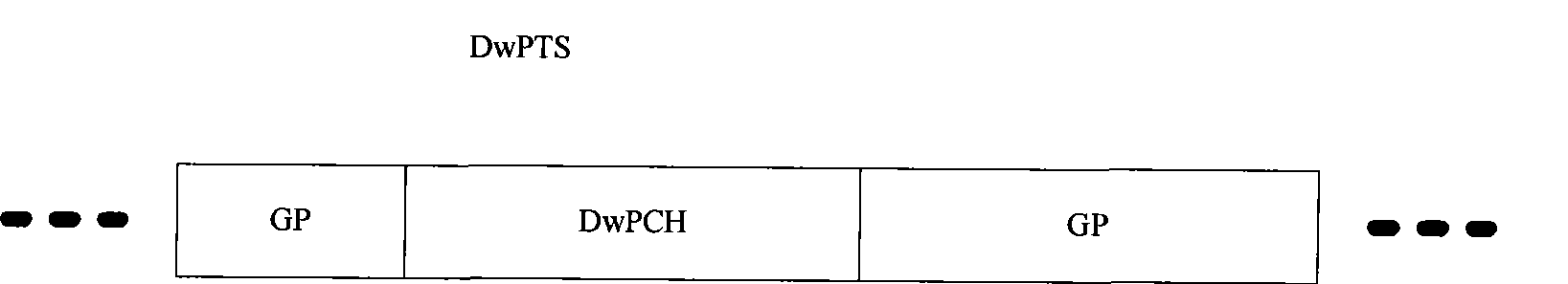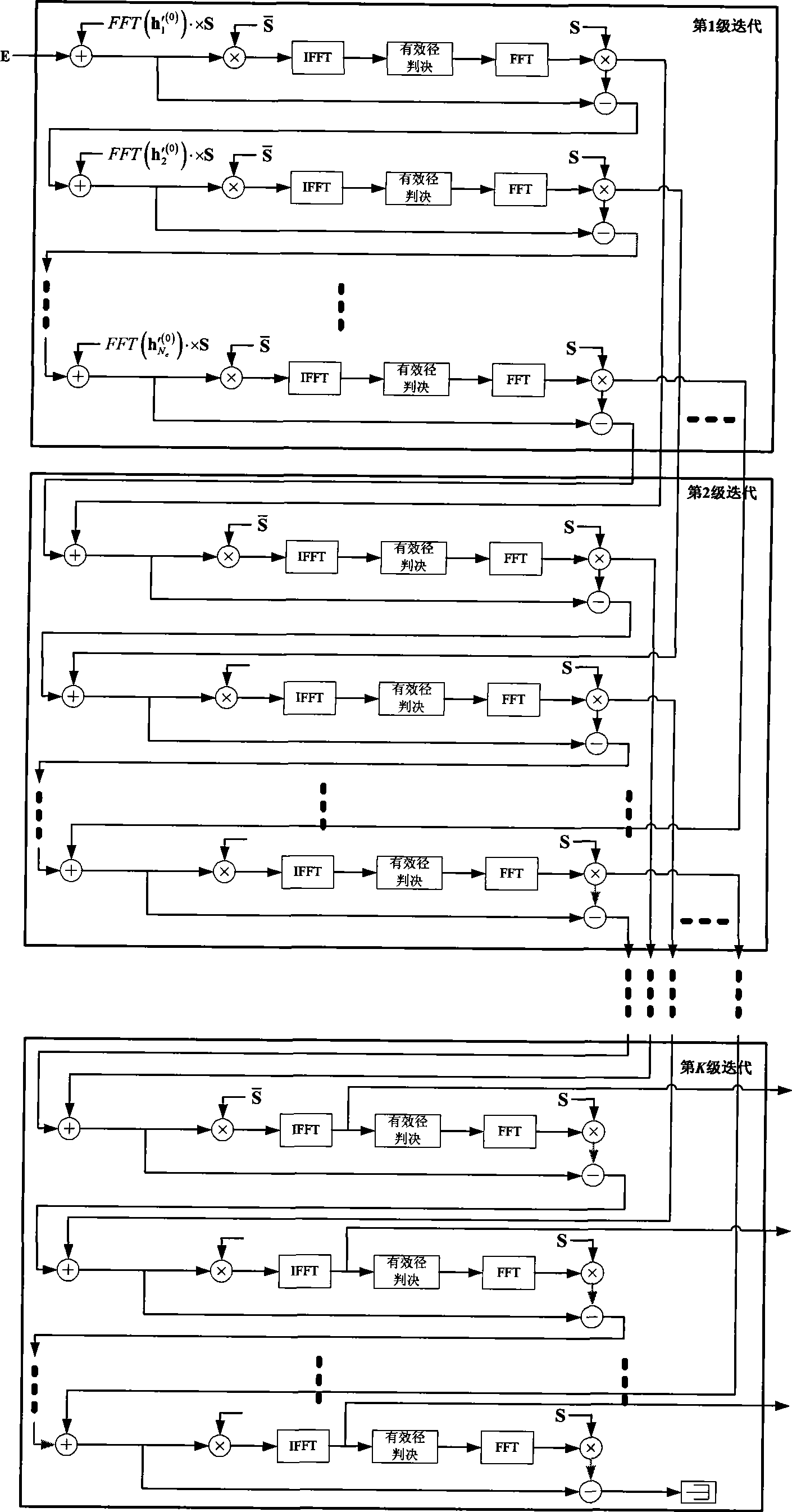# Method for measuring cofrequency multi-district observation time difference

## A measurement method and multi-cell technology, applied in the field of TD-SCDMA communication, can solve problems such as poor results and inability to meet performance requirements

Inactive Publication Date: 2009-05-27
MEDIATEK INC
0 Cites 1 Cited by

## AI-Extracted Technical Summary

### Problems solved by technology

However, in the same-frequency networking environment, especially when the UE is at the border of multiple adjacent cells, the interference signals fro...
View more

## Abstract

A measuring method for observation time difference of same frequency and multiple cells is characterized in that observation time difference of every adjacent cell is acquired by calculating channel impact response of every cell, channel impact response peak position of every cell and OTD average value of every adjacent cell by utilizing received DwPTS information from local cell, SYNC-DL code configuration information of adjacent cell acquired by broadcasting information of local cell, single cell channel estimation, effective path judgement threshold calculation, SUC ranking, SUC calculation, especially when UE is positioned at border of multi-adjacent cells, UE accurately measures the observation time difference for multi-cells of same frequency. The invention solves problem that traditional measurement has bad result which can not satisfy demand of performance, and provides technical support for widely generalization of third generation TD-SCDMA communication system.

Application Domain

Wireless communication

Technology Topic

Technical supportPeak value +5

## Image

•••## Examples

• Experimental program(1)

### Example Embodiment

 Such as figure 2 The present invention shown includes a 6-step implementation process, and the specific steps are as follows:
 Suppose there are N c For each cell (including this cell), the downlink pilot sequence corresponding to each cell uses si , I=1, 2,...N c Said, first of all, the following formula:
 s ‾ = s ‾ ( 1 ) = s * ( 1 ) s ‾ ( i ) = 0 i = 2 ~ 65 s ‾ ( i ) = s * ( 130 - i ) i = 66 ~ 128 - - - ( 1 )
 S ‾ = FFT ( s ‾ ) 64
 E=FFT(e) (2)
 H=S.×E
 h=IFFT(H)
 To estimate the channel impulse response h of each cell separately i，i =1, 2, LN c.
 Use the following method to calculate the effective path discrimination threshold for each cell:
 1) Find the power of each tap of the channel impulse response
 Ph i (j)=|h i (j)| 2 (5)
 2) Find the smallest L for all tap power (that is, for all i and j) c ·N c The effective path discrimination threshold δ can be obtained by averaging. Considering that the number of distinguishable taps of the multipath channel is generally less than 8, usually choose L c = 128-8.
 Based on the principle of serial interference cancellation to accurately solve the channel impulse response of each cell, it is necessary to sort the serial interference cancellation order of each cell, and the sorting method is as follows
 1) Find out Ph i (j) i=1~N c , J = the largest L·N from 1 to 128 c Values
 2) Calculate the above L·N c The sum of the powers belonging to different cells among the values
 3) Arrange the above power sums of each cell in descending order, and the arrangement order is the sequence of the serial interference cancellation of the cells.
 After getting the serial interference cancellation order of the cell (the joining order is from 1 to N c ), perform multi-stage serial interference cancellation to accurately solve the channel impulse response of each cell. First, solve for each cell
 s i ′ = s i O - - - ( 6 )
 S i =FFT(s′)
 Where O is a 64×1 dimensional all-zero vector.
 Then perform serial interference cancellation for each cell, where the serial interference cancellation process of the i-th cell corresponding to the k-th level is as follows
 1) Restore the i-th cell information
 E = E + FFT ( h i ′ ( k - 1 ) ) · × S - - - ( 7 )
 2) Find the channel impulse response of the i-th cell
 h i ( k ) = IFFT ( S ‾ · × E ) - - - ( 8 )
 3) Calculation Power of each tap, reserved The 8 paths with the highest medium power, judge the power of these 8 paths and the effective path decision threshold β (k) ·The size of δ, keep the path that exceeds the threshold, and set the path below the threshold to 0 to obtain β (k) In order to determine the threshold weight value, its initial setting is relatively high, and it gradually decreases as k increases.
 4) After removing the interference caused by the i-th cell, proceed to the next iteration
 E = E - FFT ( h i ′ ( k ) ) · × S - - - ( 9 )
 If it is the last stage (Kth stage) serial interference cancellation process, output the channel impulse response result h i ( K ) , i = 1 ~ N c In the formula, when k=1, there is
 h i ′ ( 0 ) = 0 i = 1 ~ N c - - - ( 10 )
 After obtaining the channel impulse response of each cell, calculate the OTD value of each neighboring cell based on the starting position of the DwPTS of the cell. The starting position of the DwPTS of this cell is the peak position of the channel impulse response of this cell, which is Assume that the peak positions of each neighboring cell are
 Update the OTD value of the i-th cell as follows
 1) if | h i ( K ) ( j max i ) | 2 β ( K ) · δ , It means that the signal of the i-th cell at the receiver is weak, so do not update the OTD value of this cell, otherwise continue to step 2)
 2) Update the OTD value of the current time slot of the neighboring cell
 OTD i = j max i - j max 1 - - - ( 11 )
 3) According to the formula OTD=(1-p)·OTD+p·OTD, perform a sliding average of the OTD value of the i-th cell to obtain the average OTD value of the cell.

## PUM## Description & Claims & Application Information

We can also present the details of the Description, Claims and Application information to help users get a comprehensive understanding of the technical details of the patent, such as background art, summary of invention, brief description of drawings, description of embodiments, and other original content. On the other hand, users can also determine the specific scope of protection of the technology through the list of claims; as well as understand the changes in the life cycle of the technology with the presentation of the patent timeline. Login to view more.
Who we serve
• R&D Engineer
• R&D Manager
• IP Professional
Why Eureka